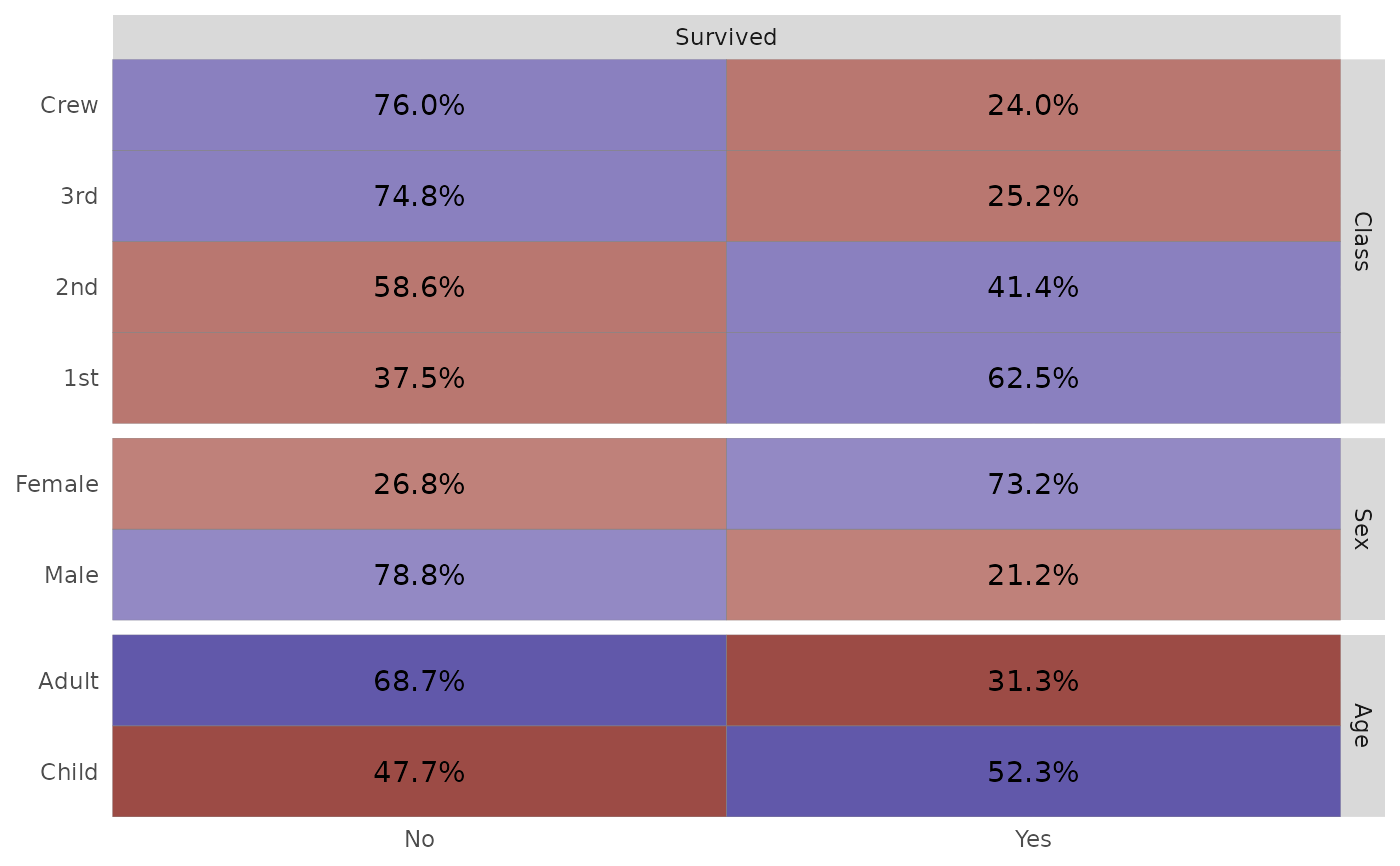ggtable is a variant of ggduo for quick cross-tabulated tables of discrete variables.

ggtable(
data,
columnsX = 1:ncol(data),
columnsY = 1:ncol(data),
cells = c("observed", "prop", "row.prop", "col.prop", "expected", "resid",
"std.resid"),
fill = c("none", "std.resid", "resid"),
mapping = NULL,
...
)

## Arguments

data dataset to be used, can have both categorical and numerical variables names or positions of which columns are used to make plots. Defaults to all columns. Which statistic should be displayed in table cells? Which statistic should be used for filling table cells? additional aesthetic to be used, for example to indicate weights (see examples) additional arguments passed to ggduo (see examples)

## Author

Joseph Larmarange

## Examples

# small function to display plots only if it's interactive
p_ <- GGally::print_if_interactive

if (require(reshape)) {
data(tips, package = "reshape")
p_(ggtable(tips, "smoker", c("day", "time", "sex")))

# displaying row proportions
p_(ggtable(tips, "smoker", c("day", "time", "sex"), cells = "row.prop"))

# filling cells with standardized residuals
p_(ggtable(tips, "smoker", c("day", "time", "sex"), fill = "std.resid", legend = 1))

# if continuous variables are provided, just displaying some summary statistics
p_(ggtable(tips, c("smoker", "total_bill"), c("day", "time", "sex", "tip")))
}
#> Loading required package: reshape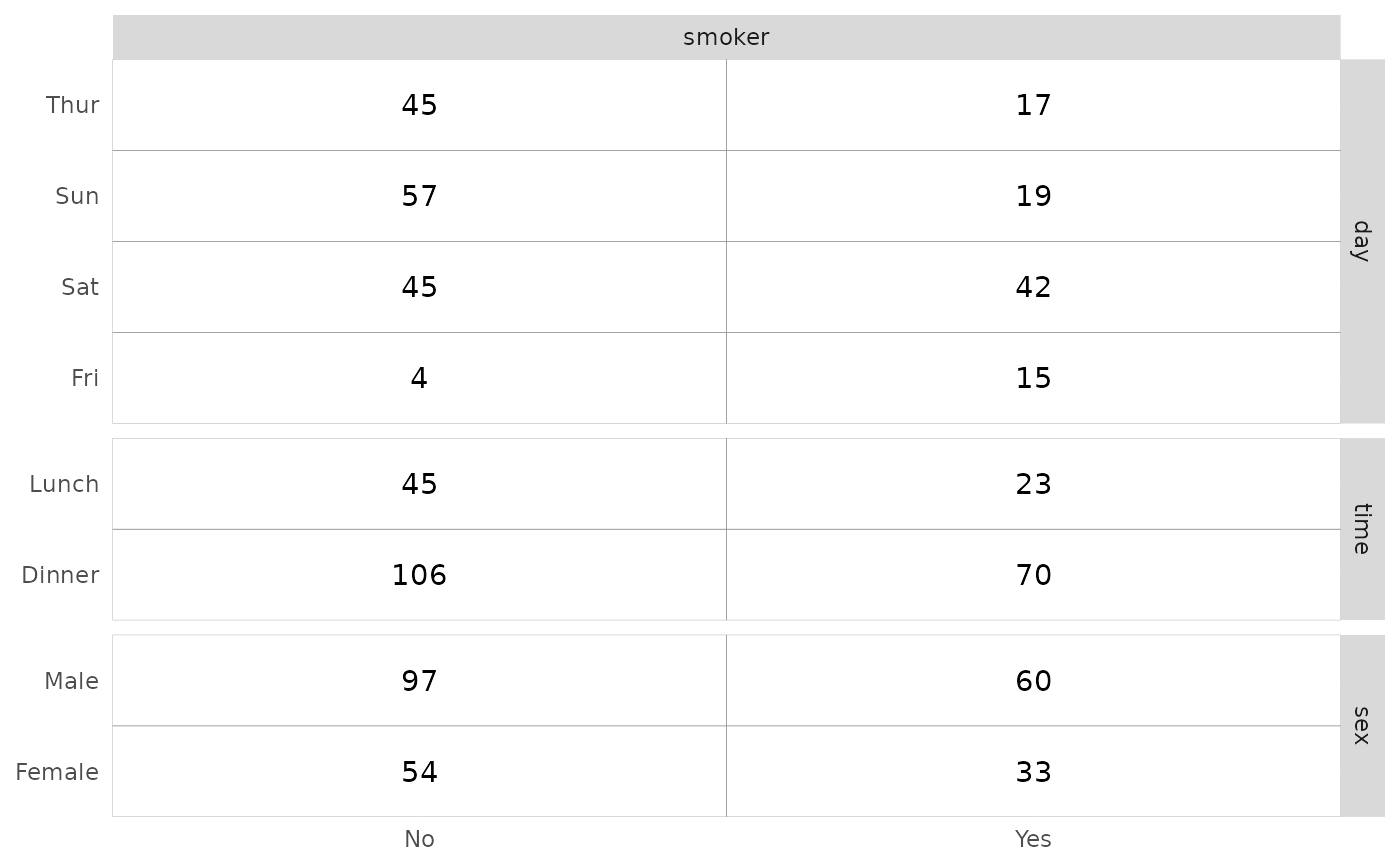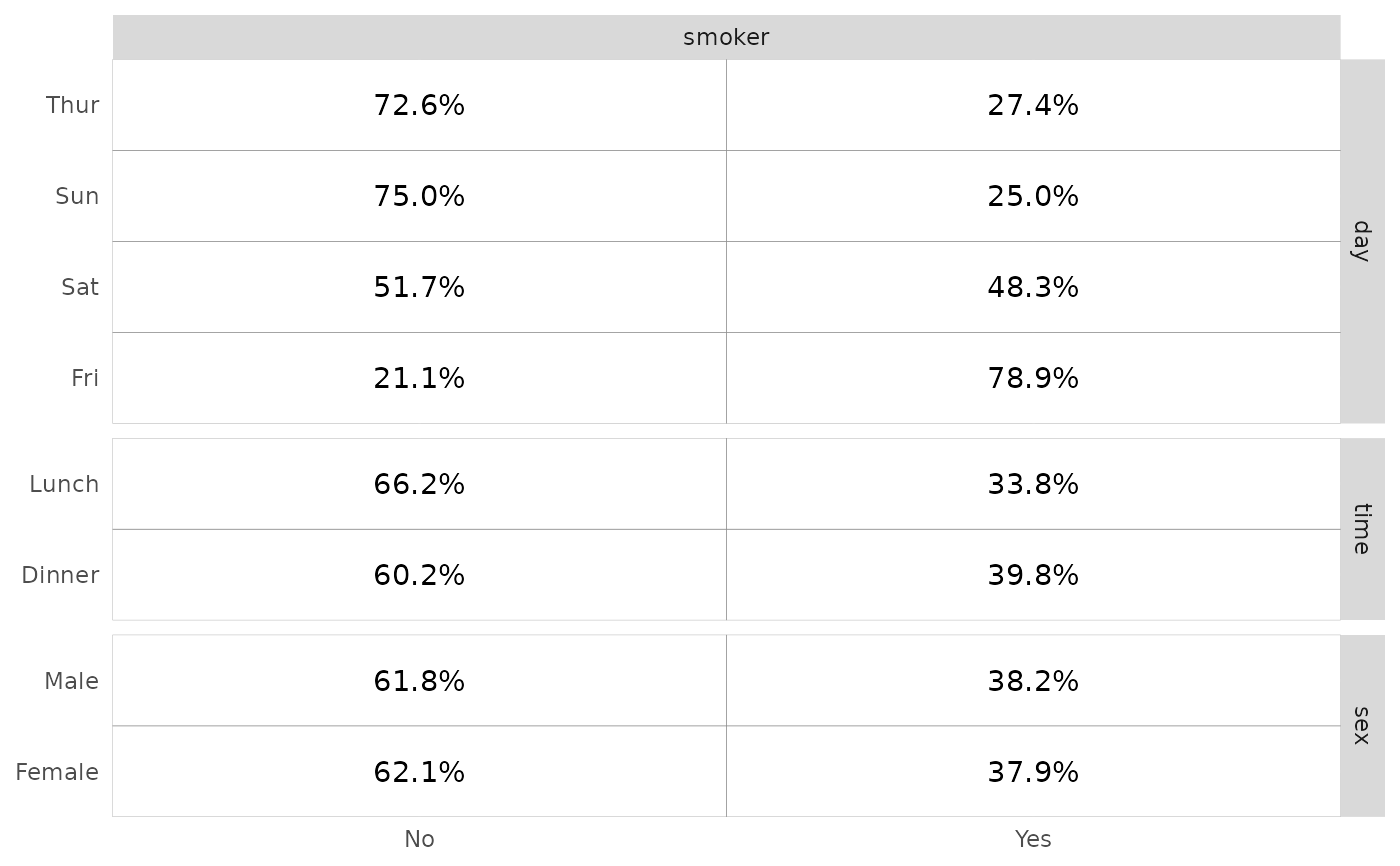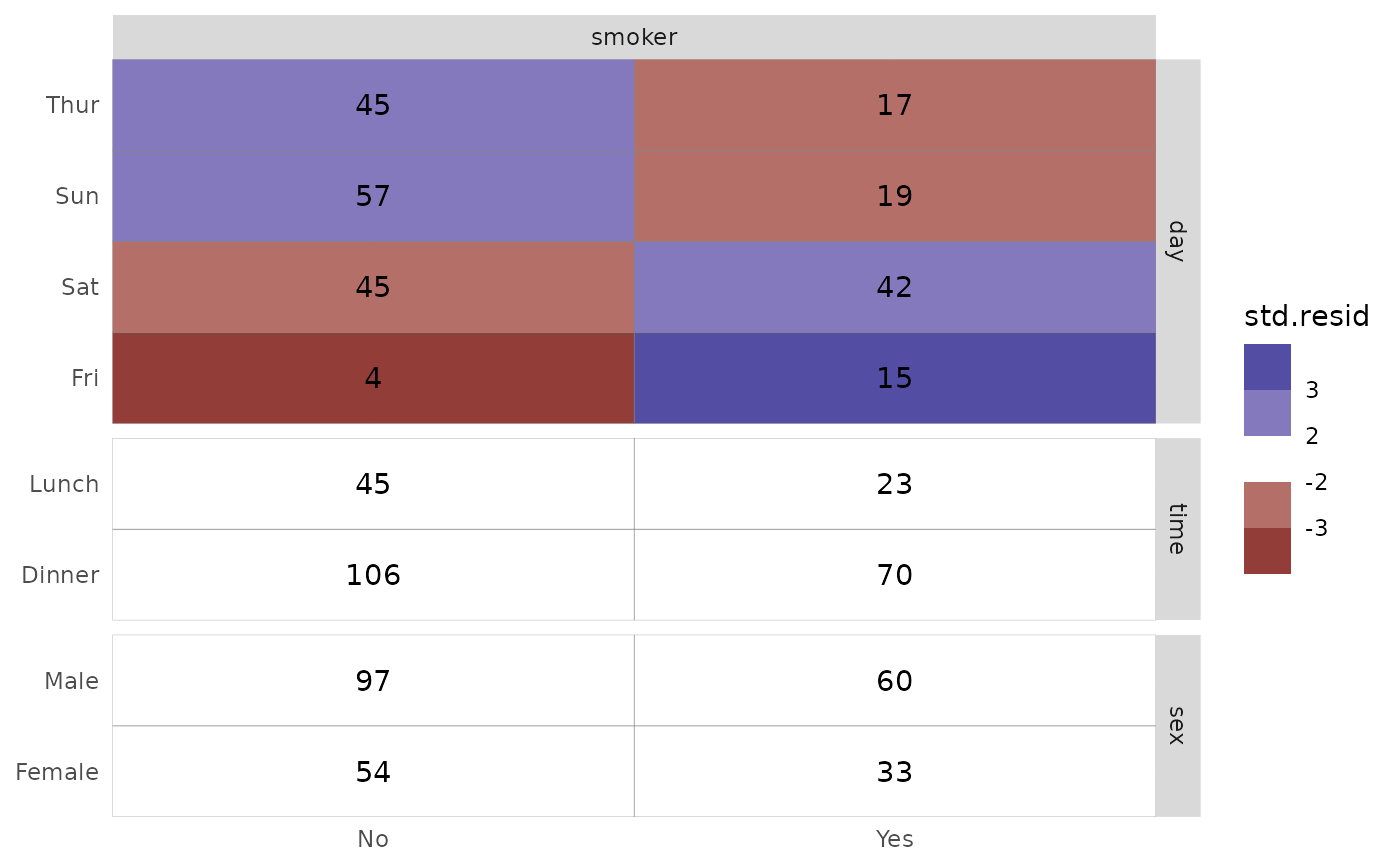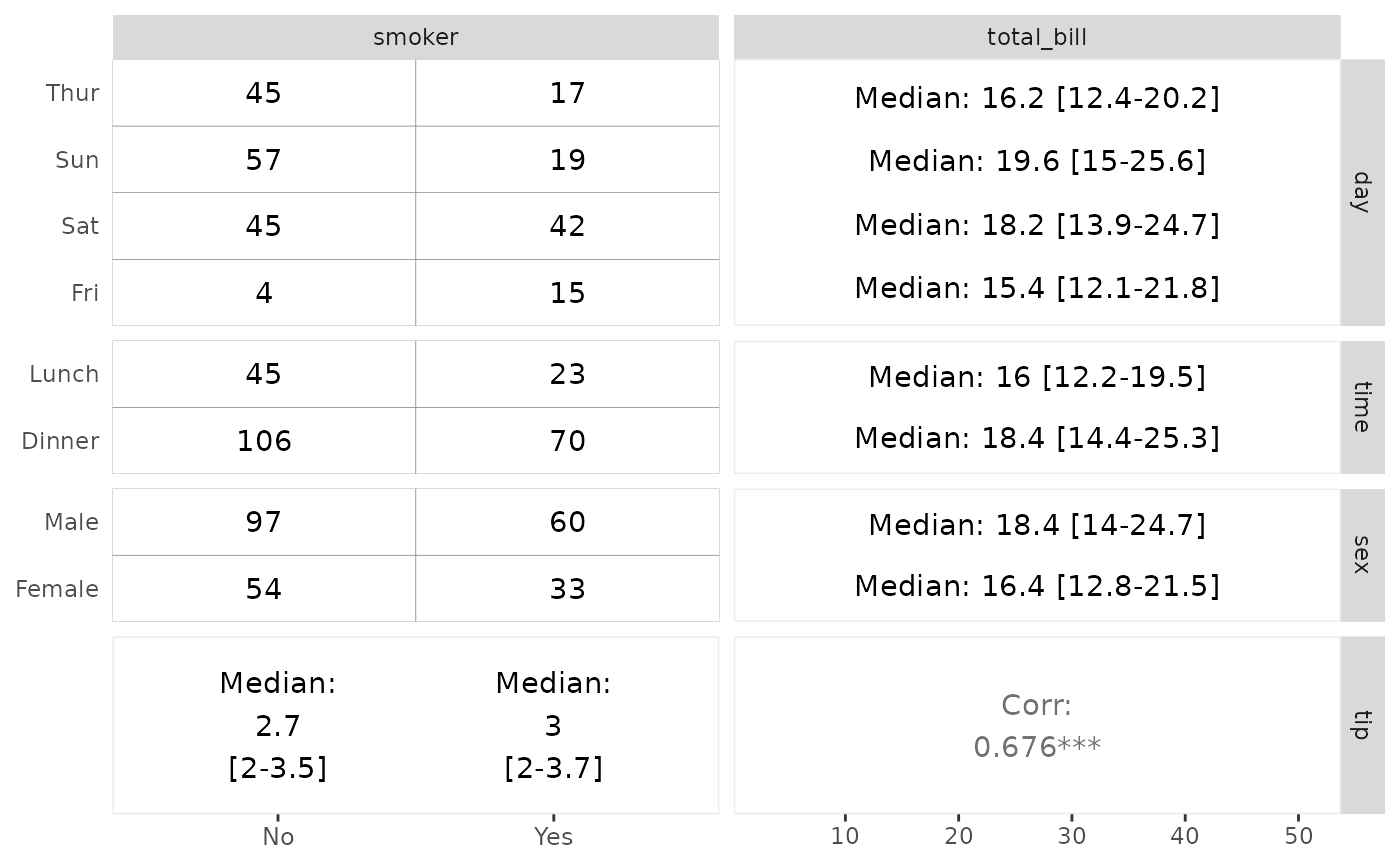# specifying weights
d <- as.data.frame(Titanic)
p_(ggtable(
d,
"Survived",
c("Class", "Sex", "Age"),
mapping = aes(weight = Freq),
cells = "row.prop",
fill = "std.resid"
))